# Search by Topic

#### Resources tagged with Simultaneous equations similar to Pareq Calc:

Filter by: Content type:
Age range:
Challenge level:

### There are 38 results

Broad Topics > Algebraic expressions, equations and formulae > Simultaneous equations### Pareq Calc

##### Age 14 to 16 Challenge Level:

Triangle ABC is an equilateral triangle with three parallel lines going through the vertices. Calculate the length of the sides of the triangle if the perpendicular distances between the parallel. . . .### Always Two

##### Age 14 to 18 Challenge Level:

Find all the triples of numbers a, b, c such that each one of them plus the product of the other two is always 2.### Leonardo's Problem

##### Age 14 to 18 Challenge Level:

A, B & C own a half, a third and a sixth of a coin collection. Each grab some coins, return some, then share equally what they had put back, finishing with their own share. How rich are they?### Polycircles

##### Age 14 to 16 Challenge Level:

Show that for any triangle it is always possible to construct 3 touching circles with centres at the vertices. Is it possible to construct touching circles centred at the vertices of any polygon?### Surds

##### Age 14 to 16 Challenge Level:

Find the exact values of x, y and a satisfying the following system of equations: 1/(a+1) = a - 1 x + y = 2a x = ay### Matchless

##### Age 14 to 16 Challenge Level:

There is a particular value of x, and a value of y to go with it, which make all five expressions equal in value, can you find that x, y pair ?### Children at Large

##### Age 11 to 14 Challenge Level:

There are four children in a family, two girls, Kate and Sally, and two boys, Tom and Ben. How old are the children?### Overturning Fracsum

##### Age 14 to 16 Challenge Level:

Solve the system of equations to find the values of x, y and z: xy/(x+y)=1/2, yz/(y+z)=1/3, zx/(z+x)=1/7### Intersections

##### Age 14 to 18 Challenge Level:

Change one equation in this pair of simultaneous equations very slightly and there is a big change in the solution. Why?### Multiplication Arithmagons

##### Age 14 to 16 Challenge Level:

Can you find the values at the vertices when you know the values on the edges of these multiplication arithmagons?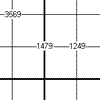### Another Quadruple Clue Sudoku

##### Age 11 to 16 Challenge Level:

This is a variation of sudoku which contains a set of special clue-numbers. Each set of 4 small digits stands for the numbers in the four cells of the grid adjacent to this set.### Arithmagons

##### Age 14 to 16 Challenge Level:

Can you find the values at the vertices when you know the values on the edges?### Fruity Totals

##### Age 7 to 16 Challenge Level:

In this interactivity each fruit has a hidden value. Can you deduce what each one is worth?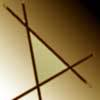### Negatively Triangular

##### Age 14 to 16 Challenge Level:

How many intersections do you expect from four straight lines ? Which three lines enclose a triangle with negative co-ordinates for every point ?### What's it Worth?

##### Age 11 to 16 Challenge Level:

There are lots of different methods to find out what the shapes are worth - how many can you find?### Gift of Gems

##### Age 14 to 16 Challenge Level:

Four jewellers share their stock. Can you work out the relative values of their gems?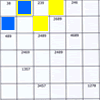### Intersection Sudoku 2

##### Age 11 to 16 Challenge Level:

A Sudoku with a twist.### Warmsnug Double Glazing

##### Age 14 to 16 Challenge Level:

How have "Warmsnug" arrived at the prices shown on their windows? Which window has been given an incorrect price?### LCM Sudoku II

##### Age 11 to 18 Challenge Level:

You are given the Lowest Common Multiples of sets of digits. Find the digits and then solve the Sudoku.### Symmetricality

##### Age 11 to 14 Challenge Level:

Add up all 5 equations given below. What do you notice? Solve the system and find the values of a, b, c , d and e. b + c + d + e = 4 a + c + d + e = 5 a + b + d + e = 1 a + b + c + e = 2 a + b. . . .### Quadruple Clue Sudoku

##### Age 11 to 16 Challenge Level:

Four numbers on an intersection that need to be placed in the surrounding cells. That is all you need to know to solve this sudoku.### All-variables Sudoku

##### Age 11 to 18 Challenge Level:

The challenge is to find the values of the variables if you are to solve this Sudoku.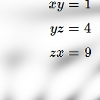### All Square

##### Age 11 to 14 Challenge Level:

Solve the system of equations xy = 1 yz = 4 zx = 9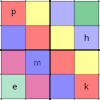### Simultaneous Equations Sudoku

##### Age 11 to 16 Challenge Level:

Solve the equations to identify the clue numbers in this Sudoku problem.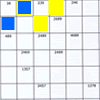### Intersection Sudoku 1

##### Age 11 to 16 Challenge Level:

A Sudoku with a twist.### CD Heaven

##### Age 14 to 16 Challenge Level:

All CD Heaven stores were given the same number of a popular CD to sell for £24. In their two week sale each store reduces the price of the CD by 25% ... How many CDs did the store sell at. . . .### Sweet Shop

##### Age 11 to 14 Challenge Level:

Five children went into the sweet shop after school. There were choco bars, chews, mini eggs and lollypops, all costing under 50p. Suggest a way in which Nathan could spend all his money.### Letter Land

##### Age 11 to 14 Challenge Level:

If: A + C = A; F x D = F; B - G = G; A + H = E; B / H = G; E - G = F and A-H represent the numbers from 0 to 7 Find the values of A, B, C, D, E, F and H.### Building Tetrahedra

##### Age 14 to 16 Challenge Level:

Can you make a tetrahedron whose faces all have the same perimeter?### Not a Polite Question

##### Age 11 to 14 Challenge Level:

When asked how old she was, the teacher replied: My age in years is not prime but odd and when reversed and added to my age you have a perfect square...### Rudolff's Problem

##### Age 14 to 16 Challenge Level:

A group of 20 people pay a total of £20 to see an exhibition. The admission price is £3 for men, £2 for women and 50p for children. How many men, women and children are there in the group?### Coffee

##### Age 14 to 16 Challenge Level:

To make 11 kilograms of this blend of coffee costs £15 per kilogram. The blend uses more Brazilian, Kenyan and Mocha coffee... How many kilograms of each type of coffee are used?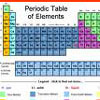### A Method of Defining Coefficients in the Equations of Chemical Reactions

##### Age 14 to 18

A simple method of defining the coefficients in the equations of chemical reactions with the help of a system of linear algebraic equations.### Which Is Bigger?

##### Age 14 to 16 Challenge Level:

Which is bigger, n+10 or 2n+3? Can you find a good method of answering similar questions?### Which Is Cheaper?

##### Age 14 to 16 Challenge Level:

When I park my car in Mathstown, there are two car parks to choose from. Can you help me to decide which one to use?### Whole Numbers Only

##### Age 11 to 14 Challenge Level:

Can you work out how many of each kind of pencil this student bought?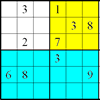### Colour Islands Sudoku

##### Age 11 to 14 Challenge Level:

An extra constraint means this Sudoku requires you to think in diagonals as well as horizontal and vertical lines and boxes of nine.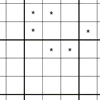### Addition Equation Sudoku

##### Age 11 to 14 Challenge Level:

You need to find the values of the stars before you can apply normal Sudoku rules.